﻿ 球形炸药水下爆炸的舰船空化效应研究
 舰船科学技术2018, Vol. 40Issue (3): 14-18PDF

Research on cavitation effect of warship underwater explosion by spherical explosive
JIA Ze, LIU Jian-bing, QUAN Lin, CHEN Gao-jie, ZHANG Shu-hong
No. 91439 Unit of PLA, Dalian 116041, China
Abstract: In this paper, we construct a finite-element model of ship, explosive and water aera through the common softwares of MSC.Dytran. After that, MSC.Dytran is employed to analyze the finite elements, and accurately simulate propagation process and cavitation effect of shock wave of underwater explosion, as well as the dynamic response of the whole ship structure. These results can serve as the input condition of anti-shock performance anlysis of ship. The numerical simulation shows that: The shock wave of spherical explosive blast propagates in the form of spherical wave, whose speed is close to the underwater velocity of sound; The relationship between instantaneous pressure of shock waves and time is exponential, which is in good agreement with Kurt theory, but with a rapid attenuation near the water due to cavitation effect. The vertical function of underwater explosion shock wave is the main factor that influence the shock response of the ship. Furthermore, the stress at the bottom of the ship is larger than that of superstructure, as it suffers most of the shock wave load, and the maximum stress concentrates in the midship meeting the blast. Explosion shock wave acts on the bottom of the ship first, and then transfers upwards through the vertical components, until reaching the deck and the superstructure of the ship. Meanwhile, the vertical components can weaken the strength of the shock movement, so the shock response of the upper components of the ship is smaller than that of the bottom or bilge, and decreases gradually .
Key words: underwater explosion     shock wave     numerical simulation     cavitation effect     dynamic response
0 引　言

1 球形炸药水下爆炸冲击载荷的理论分析

 $P = A\left( {1 - \frac{\omega }{{{R_1}V}}} \right){e^{ - {R_1}V}} + B\left( {1 - \frac{\omega }{{{R_2}V}}} \right){e^{ - {R_2}V}} + \omega \eta {\rho _0}e{\text{。}}$ (1)

 ${p_m} = {\left( {\frac{{{W^{\frac{1}{3}}}}}{R}} \right)^\alpha } \cdot k{\text{。}}$ (2)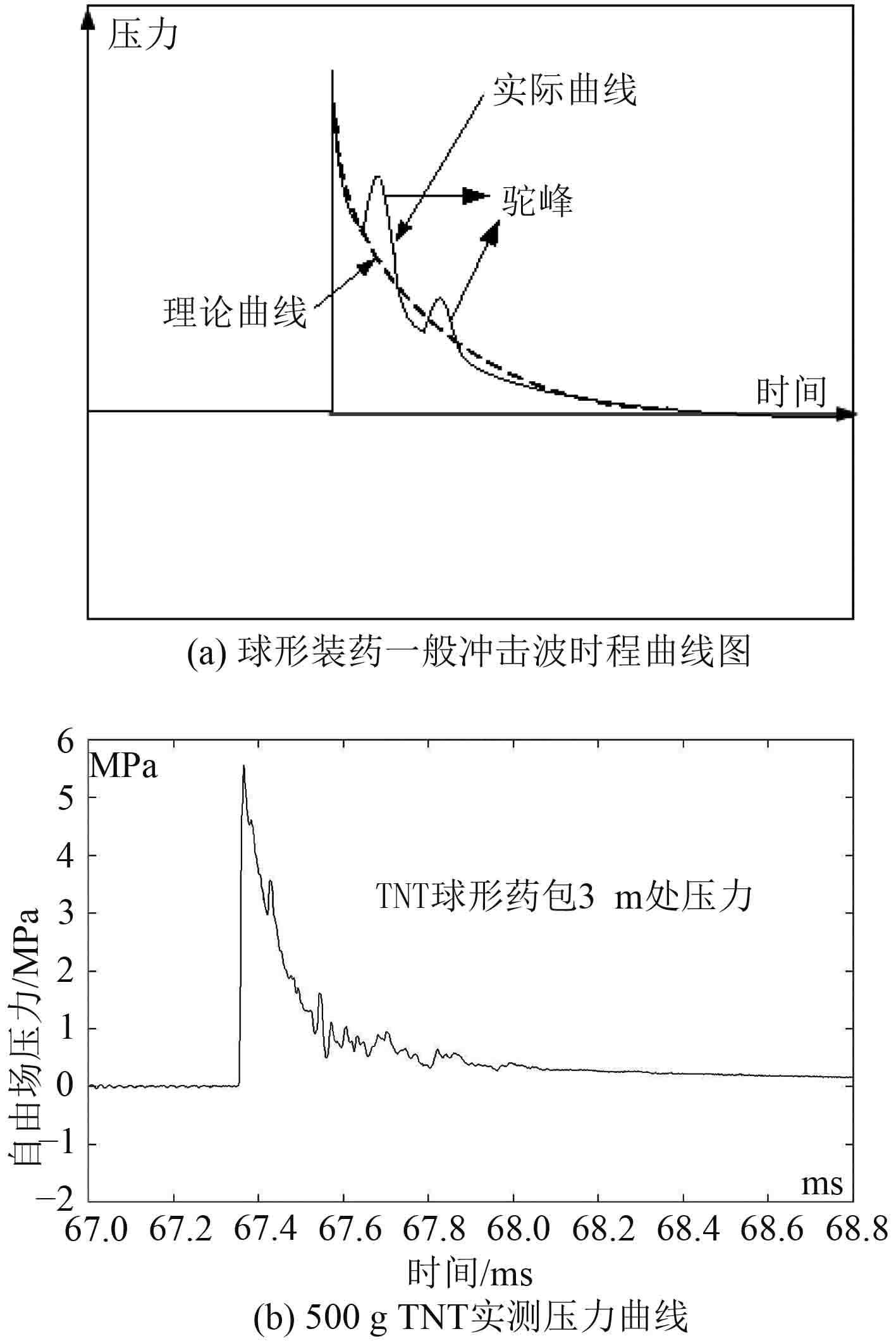图 1 球形装药冲击波时程曲线图 Fig. 1 Time-step curves of Spherical charge shock wave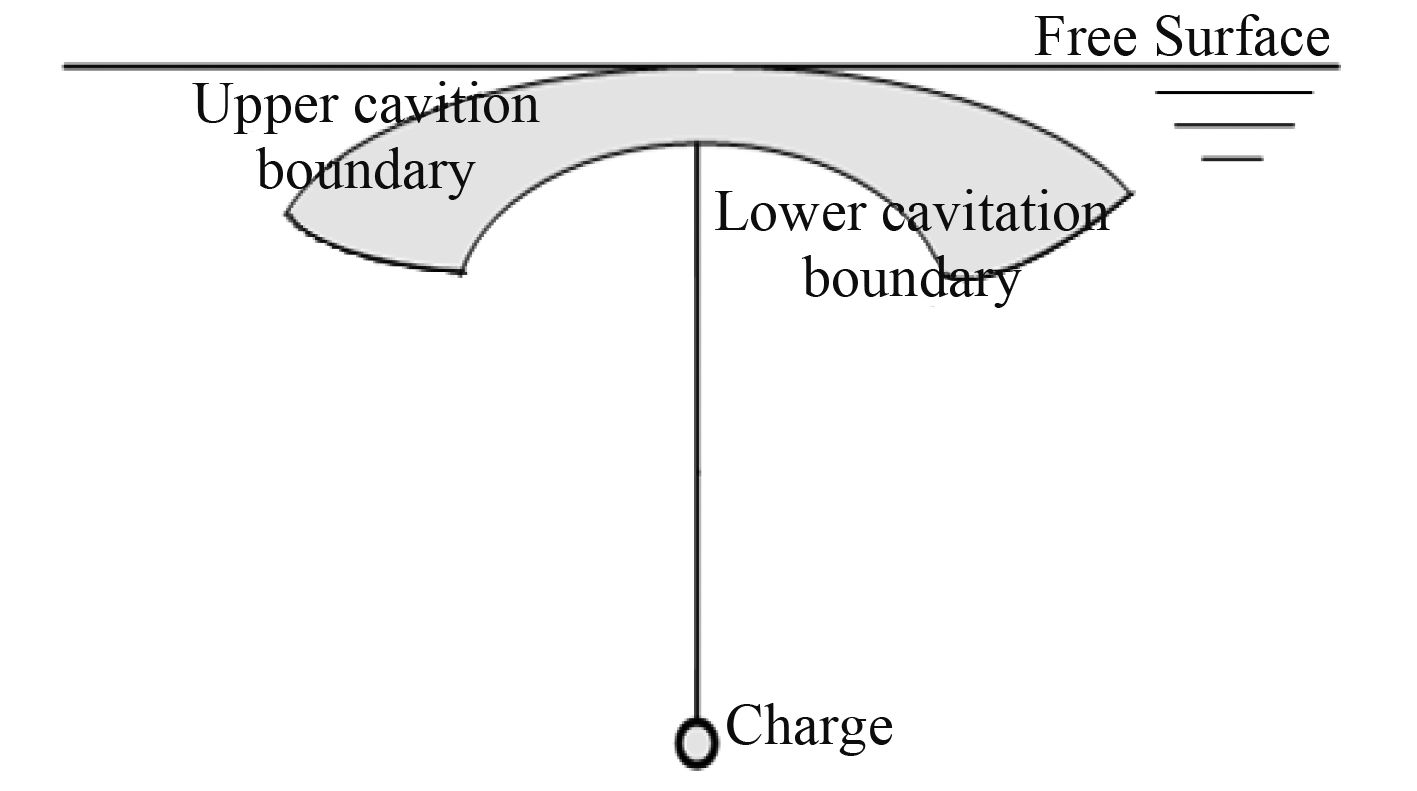图 2 水下爆炸产生的空化区域 Fig. 2 Cavitation area produced by underwater explosion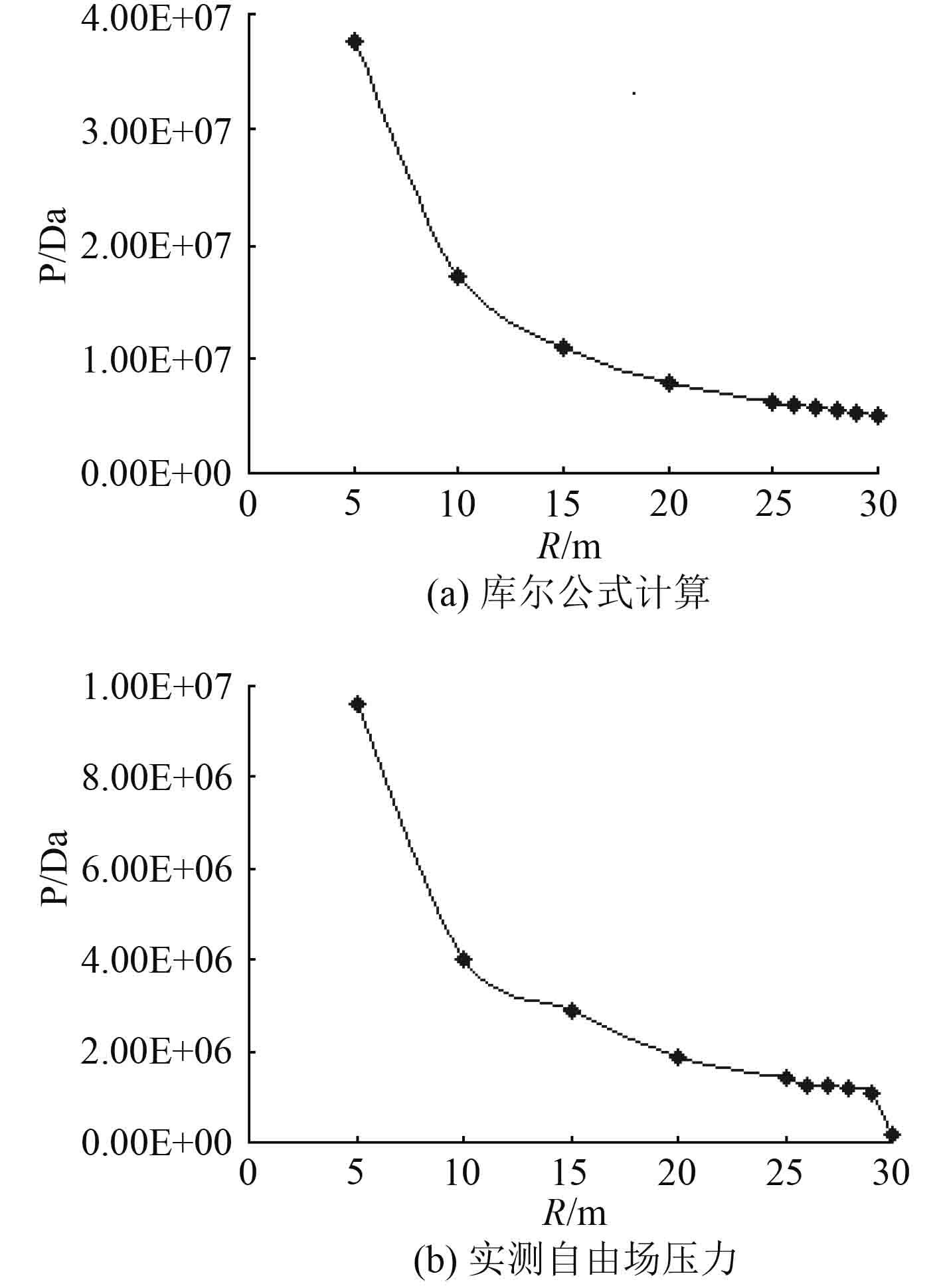图 3 冲击波压力峰值库尔公式计算结果与实测自由场压力的对比 Fig. 3 The comparison between the cole formula computing results of shock wave pressure and the measured free field pressure

2 球形炸药水下爆炸冲击载荷的数值模拟 2.1 舰船、水域和炸药有限元模型的建立 2.1.1 水域有限元模型的建模方法

 $P = B\left[ {{{\left( {\frac{\rho }{{{\rho _0}}}} \right)}^n} - 1} \right],$ (3)

 $p = {a_1}\mu + {a_2}{\mu ^2} + {a_3}{\mu ^3} + ({b_0} + {b_1}\mu + {b_2}{\mu ^2} + {b_3}{\mu ^3}){\rho _0}e{\text{。}}$ (4)

 $p = 2.18 \times {10^9}\mu + 6.69 \times {10^9}{\mu ^2} + 1.15 \times {10^{10}}{\mu ^3}{\text{。}}$ (5)

2.1.2 炸药有限元模型的建模方法

2.1.3 舰船有限元模型的建模方法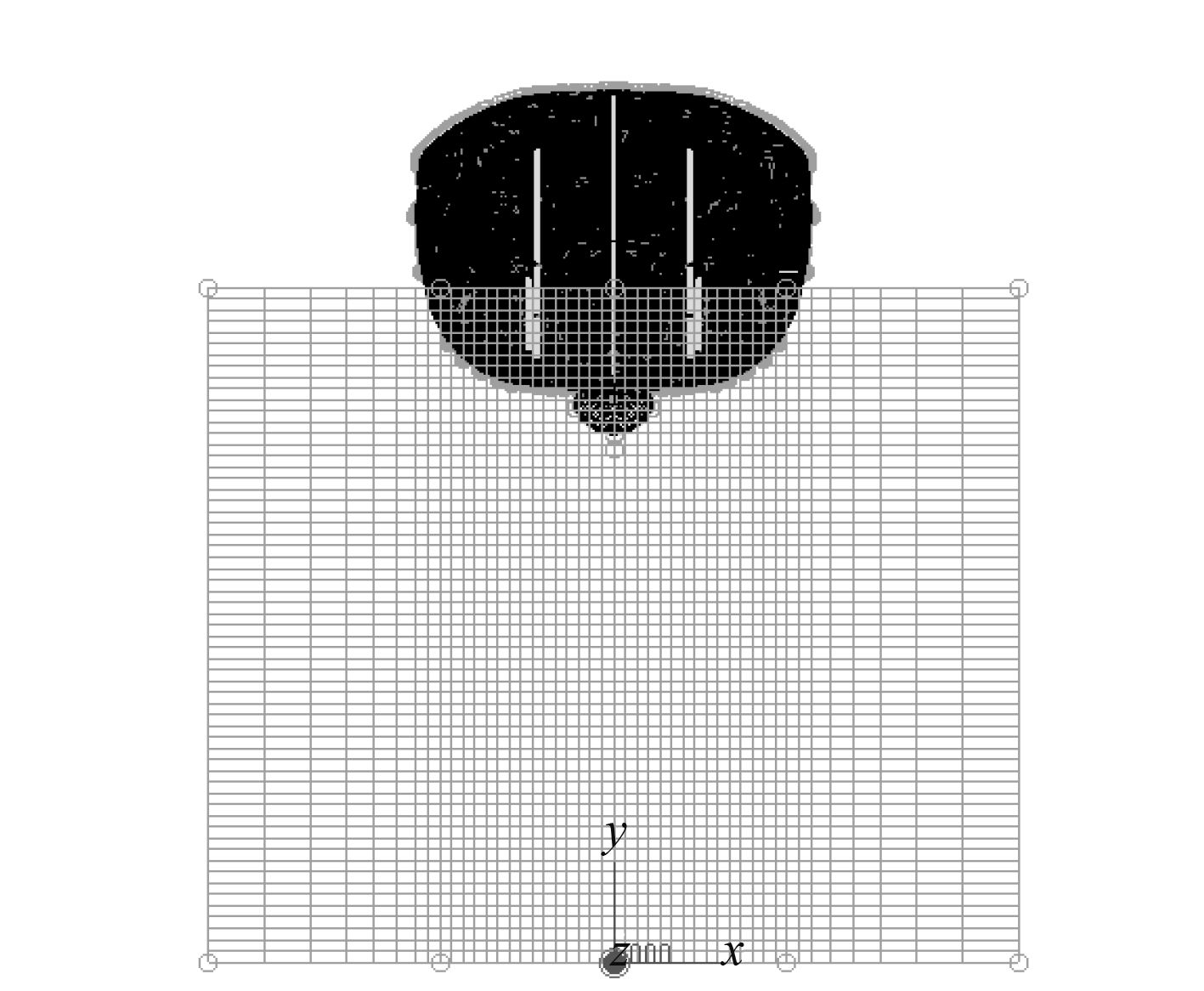图 4 舰船、水域和炸药的有限元模型 Fig. 4 Finite element models of warship, water and charge
2.2 非接触水下爆炸冲击波作用下舰船冲击响应的数值模拟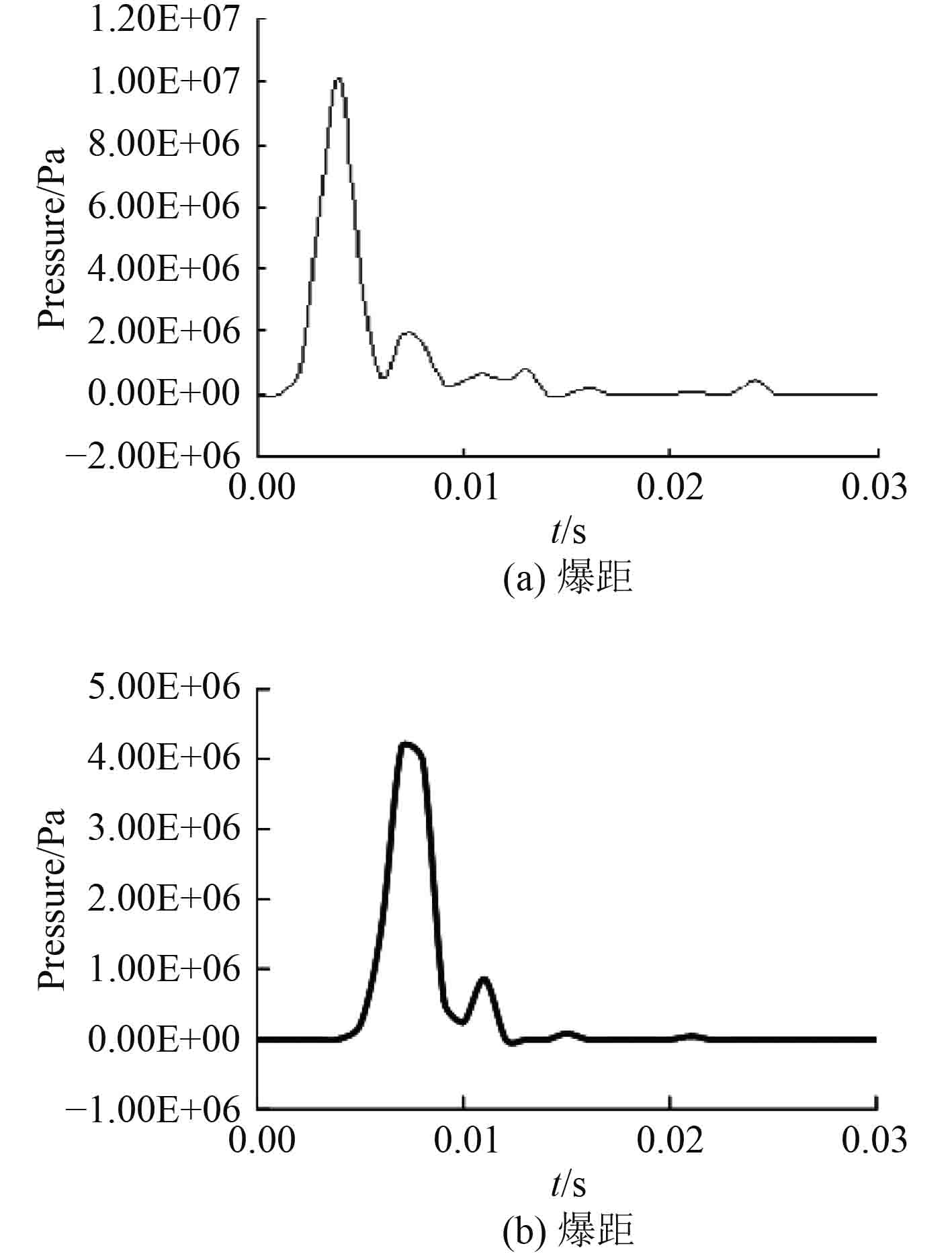图 5 水下爆炸后流场中某点的压力时历曲线图 Fig. 5 The pressure of a point in the flow field after underwater explosion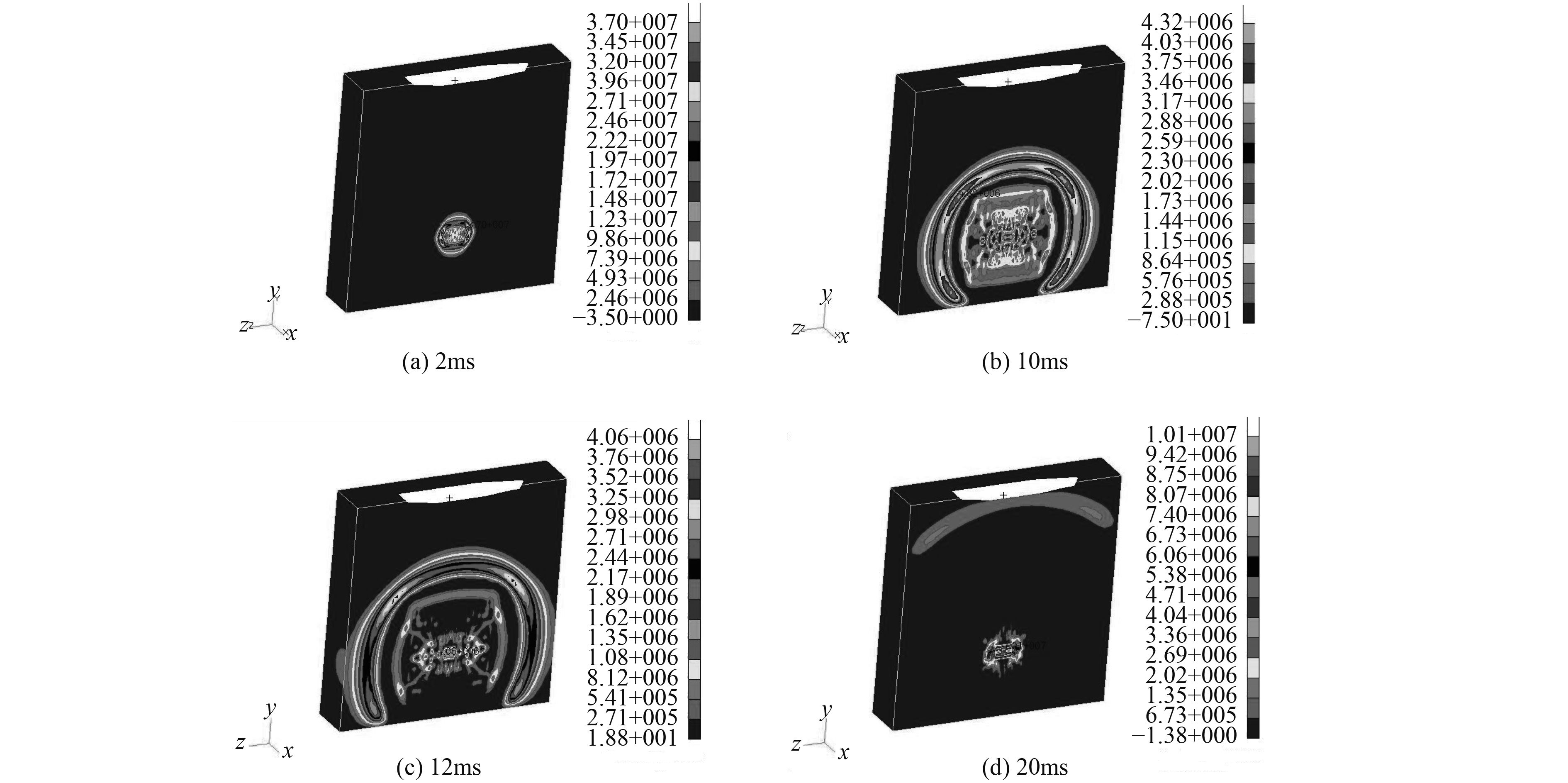图 6 水下爆炸球形冲击波扩散时历云图 Fig. 6 The spread of cloud map by the spherical shock wave of underwater explosion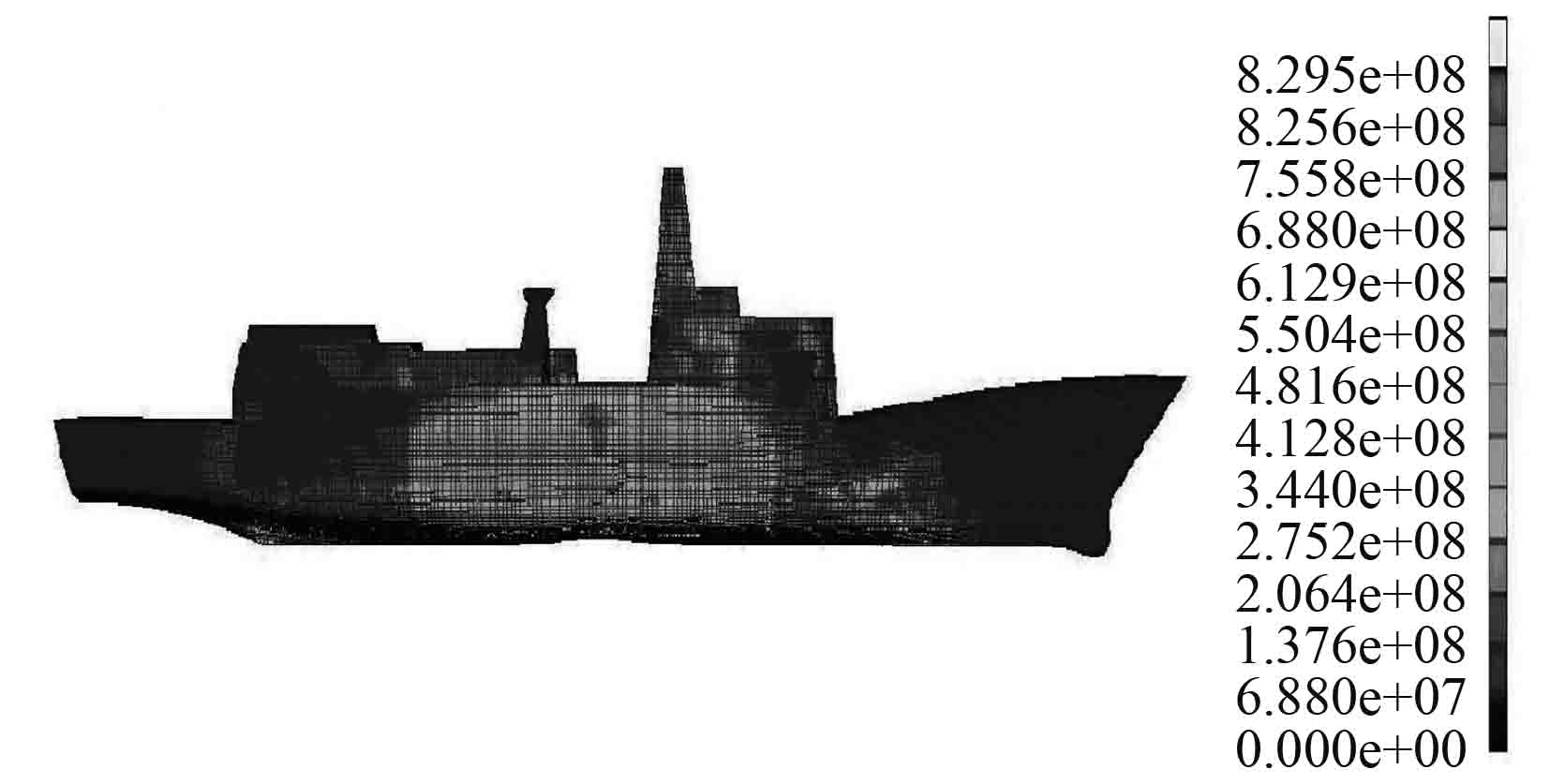图 7 水下爆炸冲击波作用下舰艇结构应力响应云图（单位：Pa） Fig. 7 The structural stress response of the warship under the action of underwater explosion shock wave

3 结　语

  陈建平. 二次脉动压力下舰船抗爆性能研究[D]. 哈尔滨: 哈尔滨工程大学. 2000, 3.  姚熊亮, 陈建平. 水下爆炸二次脉动压力下舰船抗爆性能研究[J]. 中国造船, 2001, 10(2): 48–55. http://cpfd.cnki.com.cn/Article/CPFDTOTAL-AGLU200908003040.htm  姚熊亮, 徐小刚, 张凤香. 流场网格划分对水下爆炸结构响应的影响[J]. 哈尔滨工程大学学报, 2003, 6(3): 238–244. http://d.wanfangdata.com.cn/Periodical_hebgcdxxb200303001.aspx  顾文彬, 阳天海, 叶序双, 等. 单个装药浅层水中沉底爆炸的数值模拟[J]. 解放军理工大学学报(自然科学版), 2000, 1(7): 51–55. http://d.wanfangdata.com.cn/Periodical_jfjlgdxxb200003011.aspx  ZONG Z. Dynamic plastic response of a submerged free-free beam to underwater gas bubble[J]. Acta Mechanica, 2003, 161: 179–194.  张朋祥, 顾文彬, 叶序双. 浅层水中爆炸冲击波切断现象浅探[J]. 爆炸与冲击, 2002, 7(3): 221–228. http://d.wanfangdata.com.cn/Periodical_bzycj200203006.aspx  Sang-Gab Lee. Fluid mesh modeling on surface ship shock response under underwater explosion[J]. Practical Design of Ships and other Floating Structures, 2001: 1315–1322. https://www.sciencedirect.com/science/article/pii/S0029801808000322  张振华, 朱锡, 白雪飞. 水下爆炸冲击波的数值模拟研究[J]. 爆炸与冲击, 2004, 3(2): 182–188. http://cpfd.cnki.com.cn/Article/CPFDTOTAL-AGLU201206002006.htm